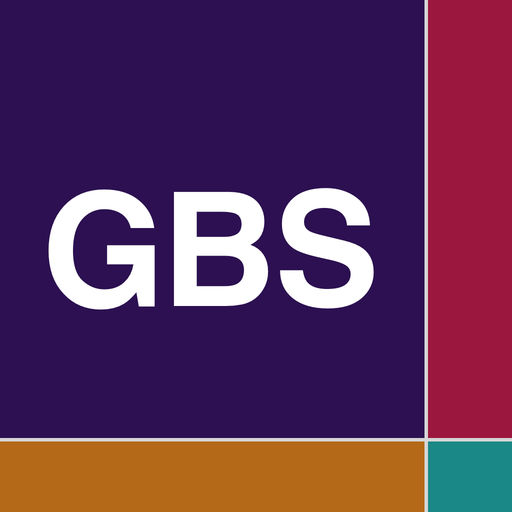Gragg-Bulirsch-Stoer Method# Gragg-Bulirsch-Stoer Method

## Donald Schaefer

1.1 for iPhone, iPad
Free
0

0 Ratings

Release Date

2019-01-23

Size

7.0 MB

### Screenshots for iPhone

iPhone
More Screenshots for iPad
Gragg-Bulirsch-Stoer Method Description
In numerical analysis, the Gragg-Bulirsch–Stoer algorithm is a method for the numerical solution of ordinary differential equations.

The Gragg-Bulirsch-Stoer method for approximating the solution of the differential equation y'(x) = f(x,y) with initial condition y = c when x = x0 is a variable step method which uses Graggs modified midpoint method for a given step size h to evaluate y(x+h), a rational function or polynomial approximation to the limit as h -> 0, and an algorithm to estimate the error based on successive estimates to y(x+h) and adjust the step size so that the absolute error is within a preassigned tolerance.

The Gragg-Bulirsch-Stoer App solves the differential equation y'=f(x,y) with the initial condition y=y0 at x. The value returned is y1 which is the is the value of y evaluated at x + h, h_new is the predicted step size so that the accuracy is maintained. The procedure terminates when the absolute difference of two successive extrapolated scaled estimates of the solution are less than epsilon.

For Data Entry the Gragg-Bulirsch-Stoer app utilizes four Sliders for the variable x0, y0, the step size(h) and the number of steps

Three graph choices display the results for the Estimate, Exact and Error Vectors The graphs are touch enabled graph. Upon touching the graph a vertical line appears. Move the vertical line to the left or right to display the data pairs for each vector

The horizontal x-axis displays x values. The vertical y-axis plots a range of Pr(x) values.

Horizontal Max and Min dashed lines display the Maximum and Minimum Pr(x) values
Price:
Free
Version:
1.1
Size:
7.0 MB
Release Date:
2019-01-23
Developer:
Donald Schaefer
Language:
English
More
You May Also Like
Developer Apps